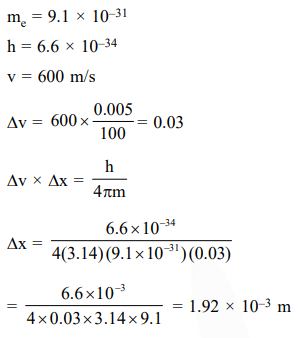# In an atom, an electron is moving with a speed

Question:

In an atom, an electron is moving with a speed of $600 \mathrm{~m} / \mathrm{s}$ with an accuracy of $0.005 \% .$ Certainity with which the position of the electron can be located is $\left(\mathrm{h}=6.6 \times 10^{-34} \mathrm{~kg} \mathrm{~m}^{2} \mathrm{~s}^{-1}\right.$, mass of electron,

$\left.\mathrm{e}_{\mathrm{m}}=9.1 \times 10^{-31} \mathrm{~kg}\right):-$

1. $1.92 \times 10^{-3} \mathrm{~m}$

2. $3.84 \times 10^{-3} \mathrm{~m}$

3. $1.52 \times 10^{-4} \mathrm{~m}$

4. $5.10 \times 10^{-3} \mathrm{~m}$

Correct Option: 1

Solution: